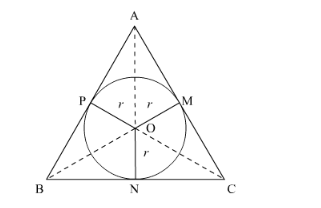# The area of the incircle of an equilateral triangle of side 42 cm isQuestion:

The area of the incircle of an equilateral triangle of side 42 cm is

(a) $22 \sqrt{3} \mathrm{~cm}^{2}$

(b) $231 \mathrm{~cm}^{2}$

(c) $462 \mathrm{~cm}^{2}$

(d) $924 \mathrm{~cm}^{2}$

Solution:

Let ABC be the equilateral triangle such that AB = BC = CA = 42 cm. Also, let O be the centre and r be the radius of its incircle.AB, BC and CA are tangents to the circle at M, N and P.

∴ OM = ON = OP = r

Area of ΔABC = Area (ΔOAB) + Area (ΔOBC) + Area (ΔOCA)

$\Rightarrow \frac{\sqrt{3}}{4}(42)^{2}=\frac{1}{2} \times r \times \mathrm{AB}+\frac{1}{2} \times r \times \mathrm{BC}+\frac{1}{2} \times r \times \mathrm{CA}$

$\Rightarrow \frac{\sqrt{3}}{4} \times 42 \times 42=\frac{1}{2} r(\mathrm{AB}+\mathrm{BC}+\mathrm{CA})$

$\Rightarrow 441 \sqrt{3}=\frac{1}{2} \times r \times(42+42+42)$

$\Rightarrow 441 \sqrt{3}=\frac{1}{2} \times r \times(126)$

$\Rightarrow 441 \sqrt{3}=63 r$

$\Rightarrow r=\frac{441 \sqrt{3}}{63}$

$\Rightarrow r=7 \sqrt{3} \mathrm{~cm}$

Area of the circle $=\pi r^{2}=\frac{22}{7}(7 \sqrt{3})^{2}=\frac{22}{7} \times 147=462 \mathrm{~cm}^{2}$

Hence, the correct answer is option (c).